Plotting Complex Sinusoids versus Frequency Next  |  Prev  |  Up  |  Top  |  Index  |  JOS Index  |  JOS Pubs  |  JOS Home  |  Search

### Plotting Complex Sinusoids versus Frequency

As discussed in the previous section, we regard the signal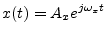as a positive-frequency sinusoid when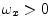. In a manner analogous to spectral magnitude plots (discussed in §4.1.6), we can plot this complex sinusoid over a frequency axis as a vertical line of length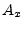at the point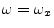, as shown in Fig.4.10. Such a plot of amplitude versus frequency may be called a spectral plot, or spectral representation  of the (zero-phase) complex sinusoid.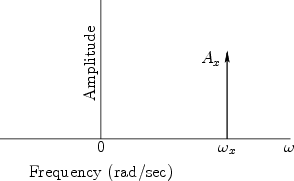More generally, however, a complex sinusoid has both an amplitude and a phase (or, equivalently, a complex amplitude):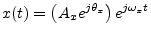To accommodate the phase angle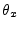in spectral plots, the plotted vector may be rotated by the anglein the plane orthogonal to the frequency axis passing through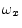, as done in Fig.4.16b below (p.) for phase angles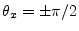.

Next  |  Prev  |  Up  |  Top  |  Index  |  JOS Index  |  JOS Pubs  |  JOS Home  |  Search

[How to cite this work] [Order a printed hardcopy]